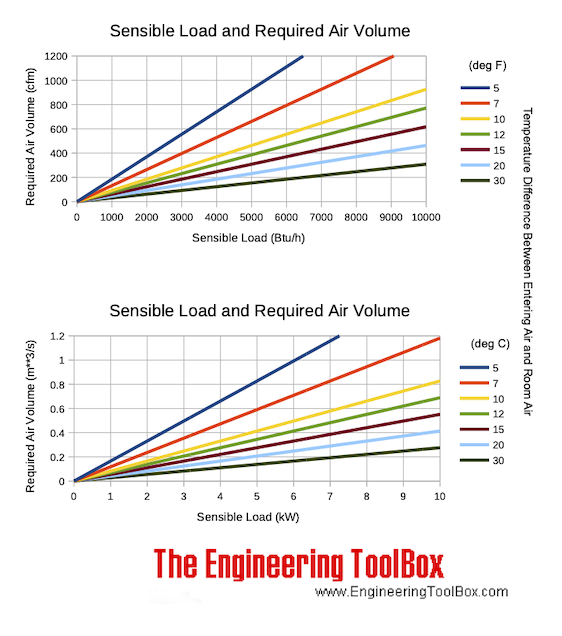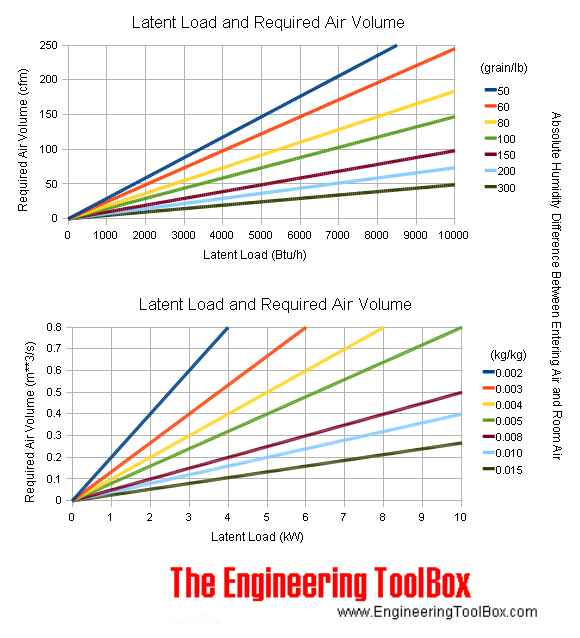Engineering ToolBox - Resources, Tools and Basic Information for Engineering and Design of Technical Applications!

# Cooling and Heating Equations

## Latent and sensible cooling and heating equations - imperial units.

### Sensible Heat

The sensible heat in a heating or cooling process of air (heating or cooling capacity) can be calculated in SI-units as

h s = c p ρ q dt (1)

where

h s = sensible heat (kW)

c p = specific heat of air (1.006 kJ/kg o C)

ρ = density of air (1.202 kg/m 3 )

q = air volume flow (m 3 /s)

dt = temperature difference ( o C)

Or in Imperial units as

h s = 1.08 q dt (1b)

where

h s = sensible heat (Btu/hr)

q = air volume flow (cfm, cubic feet per minute)

dt = temperature difference ( o F)

### Example - Heating Air, Sensible Heat

Metric Units

An air flow of 1 m 3 /s is heated from 0 to 20 o C . Using (1) the sensible heat added to the air can be calculated as

h s = (1.006 kJ/kg o C) (1.202 kg/m 3 ) ( 1 m 3 /s ) ((20 o C) - (0 o C))

= 24.2 (kW)

Imperial Units

An air flow of 1 cfm is heated from 32 to 52 o F . Using (1b) the sensible heat added to the air can be calculated as

h s = 1.08 (1 cfm) ((52 o F) - (32 o F))

= 21.6 (Btu/hr)

#### Sensible Heat Load and Required Air Volume Chart

Sensible heat load and required air volume to keep the temperature constant at various temperature differences between make up air and room air:### Latent Heat

Latent heat due to the moisture in air can be calculated in SI-units as:

h l = ρ h we q dw kg (2)

where

h l = latent heat (kW)

ρ = density of air (1.202 kg/m 3 )

q = air volume flow (m 3 /s)

h we = latent heat evaporization water ( 2454 kJ/kg - in air at atmospheric pressure and 20 o C)

dw kg = humidity ratio difference (kg water/kg dry air)

Latent evaporation heat for water can be calculated as

h we = 2494 - 2.2 t                  (2a)

where

t = evaporation temperature ( o C)

Or for Imperial units:

h l = 0.68 q dw gr (2b)

or

h l = 4840 q dw lb (2c)

where

h l = latent heat (Btu/hr)

q = air volume flow (cfm, cubic feet per minute)

dw gr = humidity ratio difference (grains water/lb dry air)

dw lb = humidity ratio difference (lb water/lb dry air)

### Example - Cooling Air, Latent Heat

Metric Units

An air flow of 1 m 3 /s is cooled from 30 to 10 o C . The relative humidity of the air is 70% at the start and 100% at the end of the cooling process.

From the Mollier diagram we estimate the water content in the hot air to be 0.0187 kg water/kg dry air, and the water content in the cold air to be 0.0075 kg water/kg dry air .

Using (2) the latent heat removed from the air can be calculated as

h l = (1.202 kg/m 3 ) ( 2454 kJ/kg ) ( 1 m 3 /s ) (( 0.0187 kg water/kg dry air ) - ( 0.0075 kg water/kg dry air ))

= 34.3 (kW)

Imperial Units

An air flow of 1 cfm is cooled from 52 to 32 o F . The relative humidity of the air is 70% at the start and 100% at the end of the cooling process.

From the psychrometric chart we estimate the water content in the hot air to be 40 grains water/lb dry air , and the water content in the cold air to be 26 grains water/lb dry air .

Using (2b) the latent heat removed from the air can be calculated as

h l = 0.68 (1 cfm) (( 40 grains water/lb dry air ) - ( 26 grains water/lb dry air ))

= 9.5 (Btu/hr)

#### Latent Heat Load and Required Air Volume Chart

Latent heat load - humidifying and dehumidifying - and required air volume to keep temperature constant at various temperature differences between entering air and room air are indicated in the chart below:### Total Heat - Latent and Sensible Heat

Total heat due to both temperature and moisture can be expressed in SI units as:

h t = ρ q dh                                            (3)

where

h t = total heat (kW)

q = air volume flow (m 3 /s)

ρ = density of air (1.202 kg/m 3 )

dh = enthalpy difference (kJ/kg)

Or - in imperial units:

h t = 4.7 q dh (3b)

where

h t = total heat (Btu/hr)

q = air volume flow (cfm, cubic feet per minute)

dh = enthalpy difference (btu/lb dry air)

Total heat can also be expressed as:

h t = h s + h l

= 1.08 q dt + 0.68 q dw gr (4)

### Example - Cooling or Heating Air, Total Heat

Metric Units

An air flow of 1 m 3 /s is cooled from 30 to 10 o C . The relative humidity of the air is 70% at the start and 100% at the end of the cooling process.

From the Mollier diagram we estimate the water enthalpy in the hot air to be 77 kJ/kg dry air, and the enthalpy in the cold air to be 28 kJ/kg dry air .

Using (3) the total sensible and latent heat removed from the air can be calculated as

h t = (1.202 kg/m 3 ) ( 1 m 3 /s ) (( 77 kJ/kg dry air ) - (28 kJ/kg dry air ))

= 58.9 (kW)

Imperial Units

An air flow of 1 cfm is cooled from 52 to 32 o F . The relative humidity of the air is 70% at the start and 100% at the end of the cooling process.

From the psychrometric chart we estimate the water enthalpy in the hot air to be 18.7 Btu /lb dry air , and the enthalpy in the cold air to be 11.8 Btu /lb dry air .

Using (3b) the total sensible and latent heat removed from the air can be calculated as

h t = 4.7 (1 cfm) (( 18.7 Btu /lb dry air ) - ( 11.8 Btu /lb dry air ))

= 32.4 (Btu/hr)

### SHR - Sensible Heat Ratio

The Sensible Heat Ratio can be expressed as

SHR = h s / h t (6)

where

SHR = Sensible Heat Ratio

h s = sensible heat

h t = total heat (sensible and latent)

## Related Topics

• ### Air Conditioning

Air Conditioning systems - heating, cooling and dehumidification of indoor air for thermal comfort.

## Related Documents

• ### Air - Heating, Cooling, Mixing, Humidifying or Dehumidifying Processes

Basic air changing state processes - heating, cooling, mixing, humidifying and dehumidifying by adding steam or water - psychometric diagrams and the Mollier charts.
• ### Air - Humidifying by Adding Steam or Water

Air can be humidified by adding water or steam.
• ### Air - Humidifying with Steam, SI units

Using steam to humidify air.
• ### Air Heating Systems

Air heating buildings - heat supply vs. air flow and temperature.
• ### Arithmetic and Logarithmic Mean Temperature Difference

Arithmetic Mean Temperature Difference in Heat Exchangers - AMTD - and Logarithmic Mean Temperature Difference - LMTD - formulas with examples - Online Mean Temperature Calculator.
• ### Cooling and Heating - Performance and Efficiency Terminology

Performance and efficiency terminology related to heat pumps and air condition systems.
• ### Cooling Load - Latent and Sensible Heat

Latent and sensible cooling loads to consider when designing HVAC systems.
• ### Dehumidifiers

Classification of dehumidifiers.
• ### Electric Heating of Mass

Electric heating of an object or mass - temperature change vs. energy supplied.
• ### Great Sensible Heat Factor - GSHF

The Great Sensible Heat Factor is the ratio sensible to total heat in a cooling coil.
• ### Heat Loss from Electrical Equipment

Heat loss from electrical equipment like switch-gear, transformers and variable frequency drives.
• ### Humid Air - Heating

Enthalpy change and temperature rise when heating humid air without adding moisture.
• ### HVAC Abbreviations

Commonly used HVAC abbreviations.
• ### Latent Heat Flow

Latent heat is the heat when supplied to or removed from air results in a change in moisture content - the temperature of the air is not changed.
• ### Mixing Liquids and/or Solids - Final Temperatures

Calculate the final temperature when liquids or solids are mixed
• ### Mixing of Humid Air

The change in state wwhen mixing moist air - enthalpy, heat, temperature and specific humidity.
• ### Moist Air - the Mollier Diagram

The Mollier diagram is a graphic representation of the relationship between air temperature, moisture content and enthalpy - and is a basic design tool for building engineers and designers.
• ### Removing Heat with Air

Calculating heat removed with air by measuring the wet bulb temperature.
• ### Room Sensible Heat Factor - RSHF

Room Sensible Heat Factor - RSHF - is defined as the sensible heat load divided by the total heat load in a room
• ### Sensible Heat Flow

Sensible heat is dry heat causing change in temperature but not in moisture content
• ### Sensible Heat Ratio - SHR

The ratio Sensible Heat (or Cooling) Load to the Total Heat (or Cooling) Load.
• ### Steam Heating Air

Calculate steam heated air systems.
• ### Steam Heating Processes - Load Calculating

Calculating the amount of steam in non-flow batch and continuous flow heating processes.
• ### Steam Radiators and Convectors - Heating Capacities

Steam radiators and steam convectors - heating capacities and temperature coefficients.

## Search

Search is the most efficient way to navigate the Engineering ToolBox.

## Engineering ToolBox - SketchUp Extension - Online 3D modeling!

Add standard and customized parametric components - like flange beams, lumbers, piping, stairs and more - to your Sketchup model with the Engineering ToolBox - SketchUp Extension - enabled for use with older versions of the amazing SketchUp Make and the newer "up to date" SketchUp Pro . Add the Engineering ToolBox extension to your SketchUp Make/Pro from the Extension Warehouse !

We don't collect information from our users. More about

## Citation

• The Engineering ToolBox (2004). Cooling and Heating Equations. [online] Available at: https://www.engineeringtoolbox.com/cooling-heating-equations-d_747.html [Accessed Day Month Year].

Modify the access date according your visit.

11.23.12